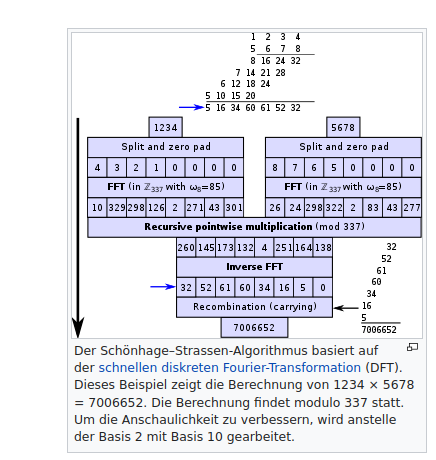# AlphaTensor takes top spot in matrix multiplication

13. October 2022

AlphaTensor takes top spot in matrix multiplication

San Francisco, Oct. 13, 2022.

Alphabet DeepMind’s new AlphaTensor AI system broke a 50-year-old record by speeding up the basic mathematical task of matrix multiplication.

This calculation is commonly used in engineering, physics simulations and even machine learning itself as a core operation in neural networks. The result could help solve problems in mathematics and increase the speed of hardware, among other things.

AlphaTensor is based on DeepMind’s AlphaZero, an AI program for board games that has already beaten human masters at chess and Go. The AI Lab describes AlphaTensor as “the first artificial intelligence system for discovering new, efficient, and provably correct algorithms.”

According to DeepMind, AlphaTensor has discovered algorithms that are more efficient than previous methods at solving various matrix sizes.

For example, the researchers trained AlphaTensor to multiply two 4×4 matrices in the fewest number of steps possible. Using reinforcement learning, the program required only 47 multiplications, outperforming the Schönhage – Strassen algorithm developed in 1971.

Normally, 64 steps are needed to multiply each row by each column.

DeepMind now plans to use AlphaTensor to search for other algorithms.

Hits: 12